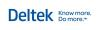You are here: Ajera Help (A-Z) > Year-end > Evaluate your year > About using fixed percentages only

# About using fixed percentages only

Fixed percentages are your best estimates of direct personnel expense (DPE) and overhead percentages. This method produces a more uniform calculation of DPE and overhead costs than the cost- and hours-based methods because it is unaffected by direct labor costs or hours.

## DPE

If you want to track DPE separately, use the following formula to calculate the percent and enter it in the Company > Preferences > Payroll tab > DPE % field. If you have different percents by department, perform the calculation for each department and enter it in the Company> Departments > DPE % field.

 Calculating DPE percent Typically, DPE percent is calculated as the total annual staff-related benefits cost divided by the total direct salary costs.    For example, if the direct salary cost is \$50,000 and the benefits cost is \$16,000, the DPE percent is: \$16,000 / \$50,000 = 32.00% Calculating DPE The DPE amount added to your project labor cost is calculated as follows: Cost Amount x DPE Percent           For example, if the cost is \$300 and DPE percent is 32.00%, then: \$300 x 32.00% = \$96

• Direct labor + DPE as the base

• Direct labor as the base

## Direct labor + DPE as the base

Use this method if you want to track your DPE separately from your general overhead expense when viewing project cost and you have entered your DPE percents.

## Direct labor as the base

Use this method if you do not want to track your DPE separately from your general overhead expense when viewing project cost.© 2015 Deltek Inc. All rights reserved.